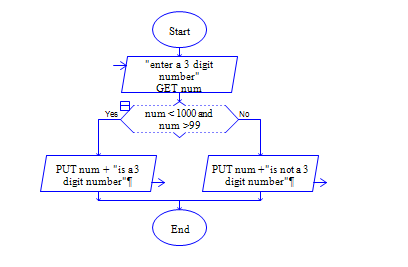# C Program to find whether the given number is 3 digit number or not

Get a number num and check whether num is three digit number or not?.

Sample Input 1:

1000

Sample Output 1:

Not a three digit number

Sample Input 2:

987

Sample Output 2:

Three digit number

#### Flow Chart Design#### Program or Solution

``` #include<stdio.h> int main() { int num; printf("Enter a number:"); scanf("%d"&num); if(num>99 && num<1000) { printf("%d is a 3 digit number",num); } else { printf("%d is not a 3 digit number",num); } return 0; } ```

#### Program Explanation

Get input num from user using scanf statement

check whether the num is greater than 99 and less than 100 using if statement.

if it is true, then print num is three digit number using printf statement.

Else print num is not three digit number using printf statement.